﻿ Number Line

Number Line

Top  Previous  Next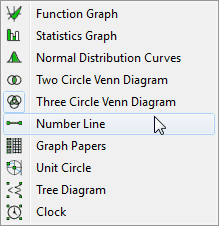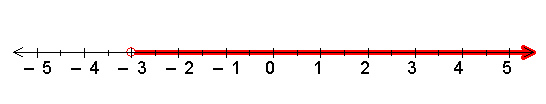To draw a number line, you sweep out a rectangle.  If the rectangle is wider than it is tall, FX Draw will create a horizontal number line.  If it is taller than it is wide, FX Draw will create a vertical number line.  When you have created the rectangle, FX Draw will create a blank number line.  To add a graph, right click on the number line.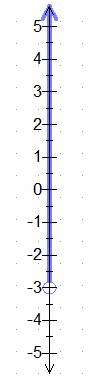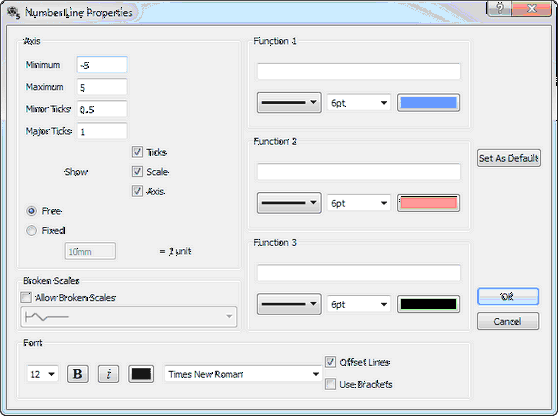This dialog box allows you to set up the axis in exactly the same way as the function graphing tool.   You enter the graphs in terms of x and you can enter the following combinations (using –3 as the number – you obviously can use any number).

x > -3                x greater than negative three

x >= -3                x greater than or equal to negative three

x < -3                x less than negative three

x <= -3                x less than or equal to negative three

x = -3                x equals negative three

x <> -3                x is not equal to negative three.

You can also place x in a range.

-3 < x <= 2        x is greater than negative three and less than or equal to two.

The Offset Lines option allows you to produce output such as this.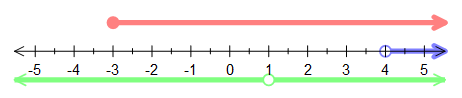Use Brackets allows you to use parentheses and square brackets instead of open and closed circles.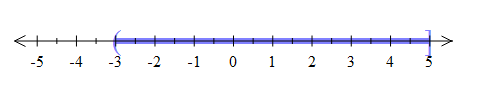Insufficient Offset

The number line tool fits the number line and any offsetted lines into the rectangle you have swept out. If your number line's offset lines are either insufficiently offset (or appear to not be offset at all), increase the size of the rectangle.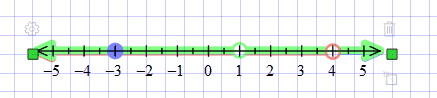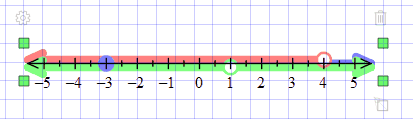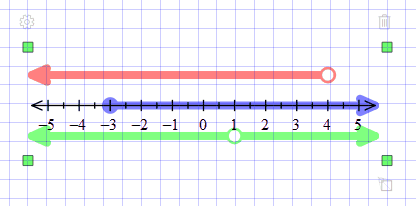Available Parameters in Number Line

\$f1                - definition of function 1

\$f2                - definition of function 2

\$f3                - definition of function 3